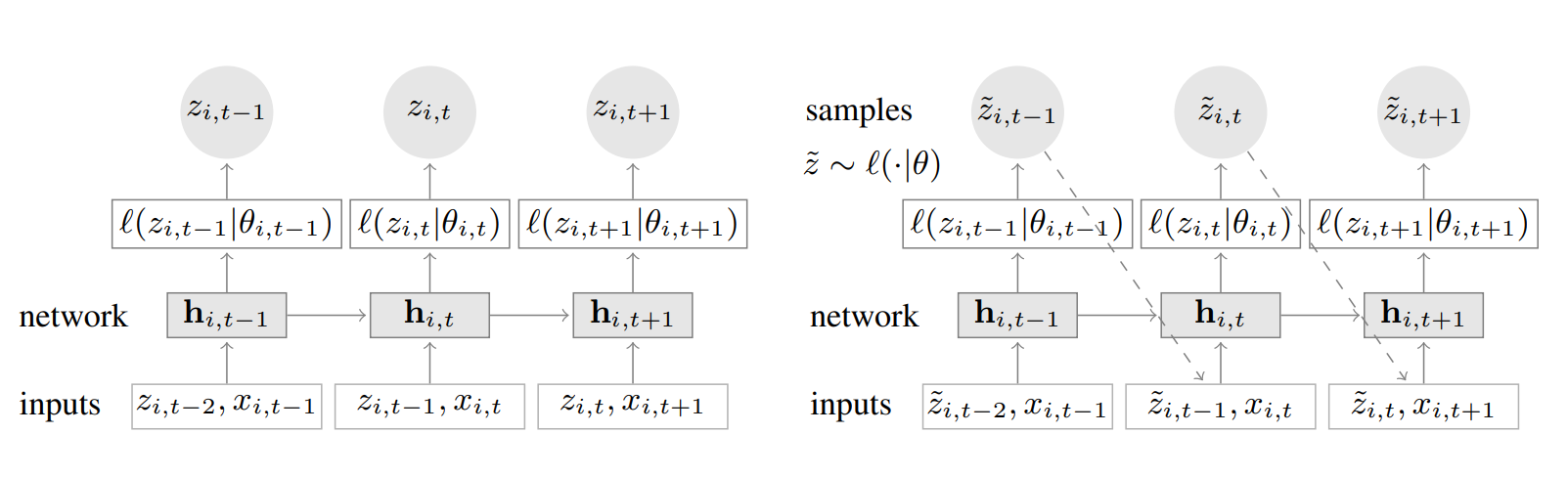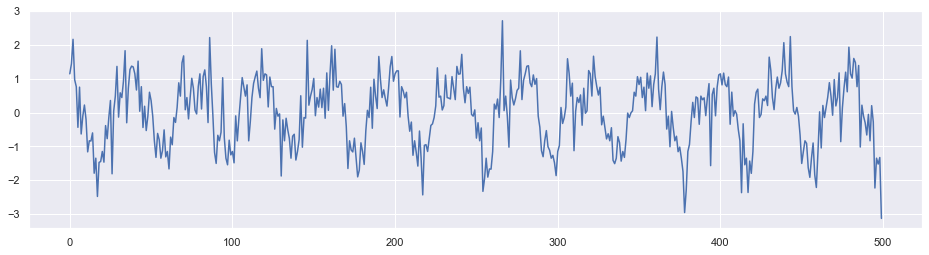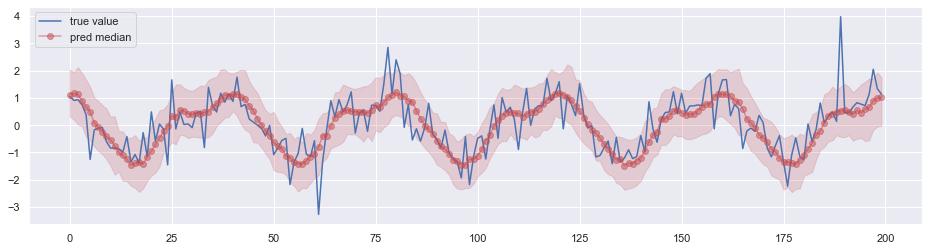DeepAR 是 Amazon 于 2017 年提出的基于深度学习的时间序列预测方法，目前已集成到 Amazon SageMakerGluonTS 中。前者是 AWS 的机器学习云平台，后者是 Amazon 开源的时序预测工具库。

## Model

$z_{i,t}$ 表示第 $i$ 个序列在时间步 $t$ 的值，$x_{i, t}$ 表示特征，$t_0$ 表示预测开始时刻。DeepAR 基于自回归循环神经网络预测 $z_{i,t}$ 的概率分布，用似然函数 $l(z_{i, t}|\theta_{i, t})$ 表示。$\mathcal L = \sum\limits_i\sum\limits_t\log l(z_{i,t}|\theta(\vec h_{i, t}))$

$\theta(\vec h_{i, t})$ 的具体形式取决于似然函数 $l(z|\theta)$，而似然函数需要根据数据本身的统计特征来选择。如果选择 Gaussian 分布，则 $\theta=(\mu, \sigma)$

\begin{aligned} l_G(z|\mu, \sigma) &= \frac{1}{\sqrt{2 \pi\sigma^2}}\exp\left(\frac{-(z- \mu)^2}{2\sigma^2}\right)\\ \mu(\vec h_{i,t}) &= \vec w_\mu^T\vec h_{i, t} + b_\mu\\ \sigma(\vec h_{i,t}) &= \log(1+\exp(\vec w_\sigma^T\vec h_{i, t} + b_\sigma)) \end{aligned}

## Code

Amazon 在 GluonTS 中提供了基于 MXNet 构建的 DeepAR 模型。由于不太熟悉 MXNet，这里提供一个基于 TensorFlow 构建的简单 demo。1. 事实上也可以有其它的预测目标，例如分位数回归↩︎